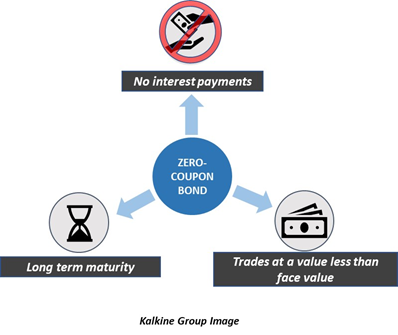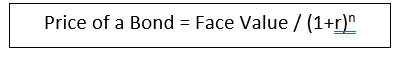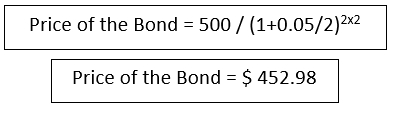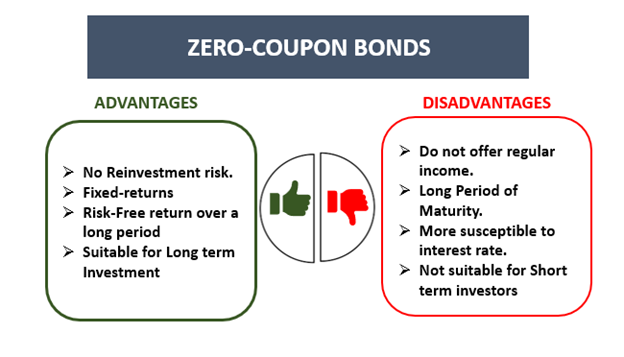# Zero-Coupon Bond

• Updated on

## What is a zero-coupon bond?

A zero-coupon bond is a bond or debt security that offers an interest rate of 0%. A zero-coupon bond offers a profit at maturity, and it trades at a lesser value than its face value. Zero-coupon bonds are also known as pure discount bonds or deep discount bonds.

The difference between the face value and the value at which it is traded allows the room for generating a profit in a zero-coupon bond. It can be understood as a long-term investment which does not fetch any interest.

Government treasury bills are examples of zero-coupon bonds. They may usually have a maturity period of about 10-15 years.  Zero-coupon bonds can be of two types: corporate zero-coupon bonds and government zero-coupon bonds.

Also Read: What are corporate bonds?## Why does a zero-coupon bond trade at a lesser value than the face value?

A zero-coupon bond does not have a periodic interest rate attached to it. The face value of a bond refers to the price of the bond when it is first purchased. The price of a bond changes after the first time it is bought. It is also known as the par value of the bond. The price is described relative to the face value of the bond.

The reason for the traded value being lesser than the face value is because of the concept of the time value of money. As time passes, the same amount of money today would hold lesser value in the future. For instance, a sum of \$50 in the current time would be of higher value than the same amount received in a year’s time instead. This happens because of the concept of the time value of money.

An investor could put his or her money in a savings deposit or in any other investment that could fetch him a greater amount than just holding the amount with him. That is why a sum of \$50 would be preferable to an investor today than in the future.

Because of this reason, an investor must be compensated with a discount on the price of the zero-coupon bond. The issuer must offer a return to the buyer for investing in the bond for such a long term.

## How is a zero-coupon bond priced?

The price of a zero-coupon bond is calculated with the following formula:Face Value is the value given at maturity of the bond, ‘r’ is the rate of interest, and ‘n’ is the number of years till maturity.

The formula above applies to bonds compounded annually. Bonds can also be compounded semi-annually.

For instance, consider a zero-coupon bond with a face value of \$500 and 2 years to maturity. The interest is annually compounded at a rate of 5%. Therefore, Price of the bond = 500/ (1.05)2

Therefore, the price of the bond becomes \$453. 514. An investor would have to pay \$453.51 to buy this bond for which the interest is compounded annually.

Now consider the case when interest is compounded semi-annually. In this case, the above formula should be modified.

The price of the bond becomes:## What are the risks associated with zero-coupon bonds?

Reinvestment risk refers to the risk that an investor would not be able to reinvest the coupon payments at a rate equal to the required rate of return offered on a bond. Zero-coupon bonds do not have reinvestment risk as there are no coupon payments. This is the only type of bond in which there is no risk of investment, and that happens because there are no coupons.

Another risk associated with bonds is the interest rate risk. It is the risk that the bond’s value would decline because of the variations in the interest rate. When an investor decides to sell a bond ahead of its maturity instead of taking the face value of the bond at maturity, a decline in the value of bonds would incur him a loss.

Zero-coupon bonds are not immune to interest rate risk. Fluctuations in the interest can cause the value of a bond to decline over time which would decrease the price of a bond in case the investor wishes to sell it ahead of maturity.

## What are the pros and cons offered by Zero-coupon bonds?

Zero-coupon bonds do not offer a regular income in the form of coupons. This might make them less preferred among certain types of investors. The long period of maturity might also make zero-coupon bonds less appealing to investors, as the profits take longer to realise. The long duration also makes these bonds more susceptible to interest rate risk.Kalkine Group Image

A zero-coupon bond's advantages include no reinvestment risk, fixed-returns, and risk-free return over a long period. These bonds may suit long-term investors and not the investors who are looking for returns in the short run.

##### Top ASX Listed Companies

Definition:
We use cookies to ensure that we give you the best experience on our website. If you continue to use this site we will assume that you are happy with it. OK